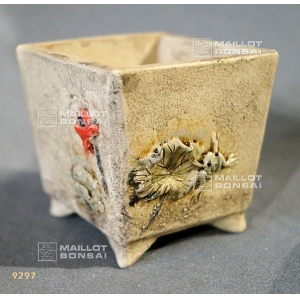##### The Japanese Bonsai specialist
Direct order Contact Help / Services Newsletter# Mini chinoiserie pot frog motif

› Bonsai pots › Mini potref. : 929728,40

Available quantity : 4Order

###### Description

Measurements of: 64*64*60 mm.Colour: ivory. An excellent present idea, this Asian vintage mini pot features a lotus leaf and frog motif in relief on the façade.It's made according to traditional methods and the sandstone is cooked at 900° over 10 hours and then a second time at 1,210° for 12 hours.

#mini 5.4 #motif 3.5 #frog 3.4 #chinoiserie 3.1 #hours 2.5 #measurements 2.2 #traditional 2.1 #according 1.9 #sandstone 1.9 #excellent 1.9

Formule
(( ROUND((CHAR_LENGTH(b.article_nom)-CHAR_LENGTH(REPLACE(b.article_nom, 'motif', '')))/LENGTH('motif')) + ROUND((CHAR_LENGTH(b.article_description)-CHAR_LENGTH(REPLACE(b.article_description, 'motif', '')))/LENGTH('motif')) ) * 3.5) + (( ROUND((CHAR_LENGTH(b.article_nom)-CHAR_LENGTH(REPLACE(b.article_nom, 'frog', '')))/LENGTH('frog')) + ROUND((CHAR_LENGTH(b.article_description)-CHAR_LENGTH(REPLACE(b.article_description, 'frog', '')))/LENGTH('frog')) ) * 3.4) + (( ROUND((CHAR_LENGTH(b.article_nom)-CHAR_LENGTH(REPLACE(b.article_nom, 'mini', '')))/LENGTH('mini')) + ROUND((CHAR_LENGTH(b.article_description)-CHAR_LENGTH(REPLACE(b.article_description, 'mini', '')))/LENGTH('mini')) ) * 3.4) + (( ROUND((CHAR_LENGTH(b.article_nom)-CHAR_LENGTH(REPLACE(b.article_nom, 'chinoiserie', '')))/LENGTH('chinoiserie')) + ROUND((CHAR_LENGTH(b.article_description)-CHAR_LENGTH(REPLACE(b.article_description, 'chinoiserie', '')))/LENGTH('chinoiserie')) ) * 3.1) + (( ROUND((CHAR_LENGTH(b.article_nom)-CHAR_LENGTH(REPLACE(b.article_nom, 'hours', '')))/LENGTH('hours')) + ROUND((CHAR_LENGTH(b.article_description)-CHAR_LENGTH(REPLACE(b.article_description, 'hours', '')))/LENGTH('hours')) ) * 2.5) + (( ROUND((CHAR_LENGTH(b.article_nom)-CHAR_LENGTH(REPLACE(b.article_nom, 'measurements', '')))/LENGTH('measurements')) + ROUND((CHAR_LENGTH(b.article_description)-CHAR_LENGTH(REPLACE(b.article_description, 'measurements', '')))/LENGTH('measurements')) ) * 2.2) + (( ROUND((CHAR_LENGTH(b.article_nom)-CHAR_LENGTH(REPLACE(b.article_nom, 'traditional', '')))/LENGTH('traditional')) + ROUND((CHAR_LENGTH(b.article_description)-CHAR_LENGTH(REPLACE(b.article_description, 'traditional', '')))/LENGTH('traditional')) ) * 2.1) + (( ROUND((CHAR_LENGTH(b.article_nom)-CHAR_LENGTH(REPLACE(b.article_nom, 'according', '')))/LENGTH('according')) + ROUND((CHAR_LENGTH(b.article_description)-CHAR_LENGTH(REPLACE(b.article_description, 'according', '')))/LENGTH('according')) ) * 1.9) + (( ROUND((CHAR_LENGTH(b.article_nom)-CHAR_LENGTH(REPLACE(b.article_nom, 'sandstone', '')))/LENGTH('sandstone')) + ROUND((CHAR_LENGTH(b.article_description)-CHAR_LENGTH(REPLACE(b.article_description, 'sandstone', '')))/LENGTH('sandstone')) ) * 1.9) + (( ROUND((CHAR_LENGTH(b.article_nom)-CHAR_LENGTH(REPLACE(b.article_nom, 'excellent', '')))/LENGTH('excellent')) + ROUND((CHAR_LENGTH(b.article_description)-CHAR_LENGTH(REPLACE(b.article_description, 'excellent', '')))/LENGTH('excellent')) ) * 1.9)

## Secure payment## Delivery

Our logistic partners :